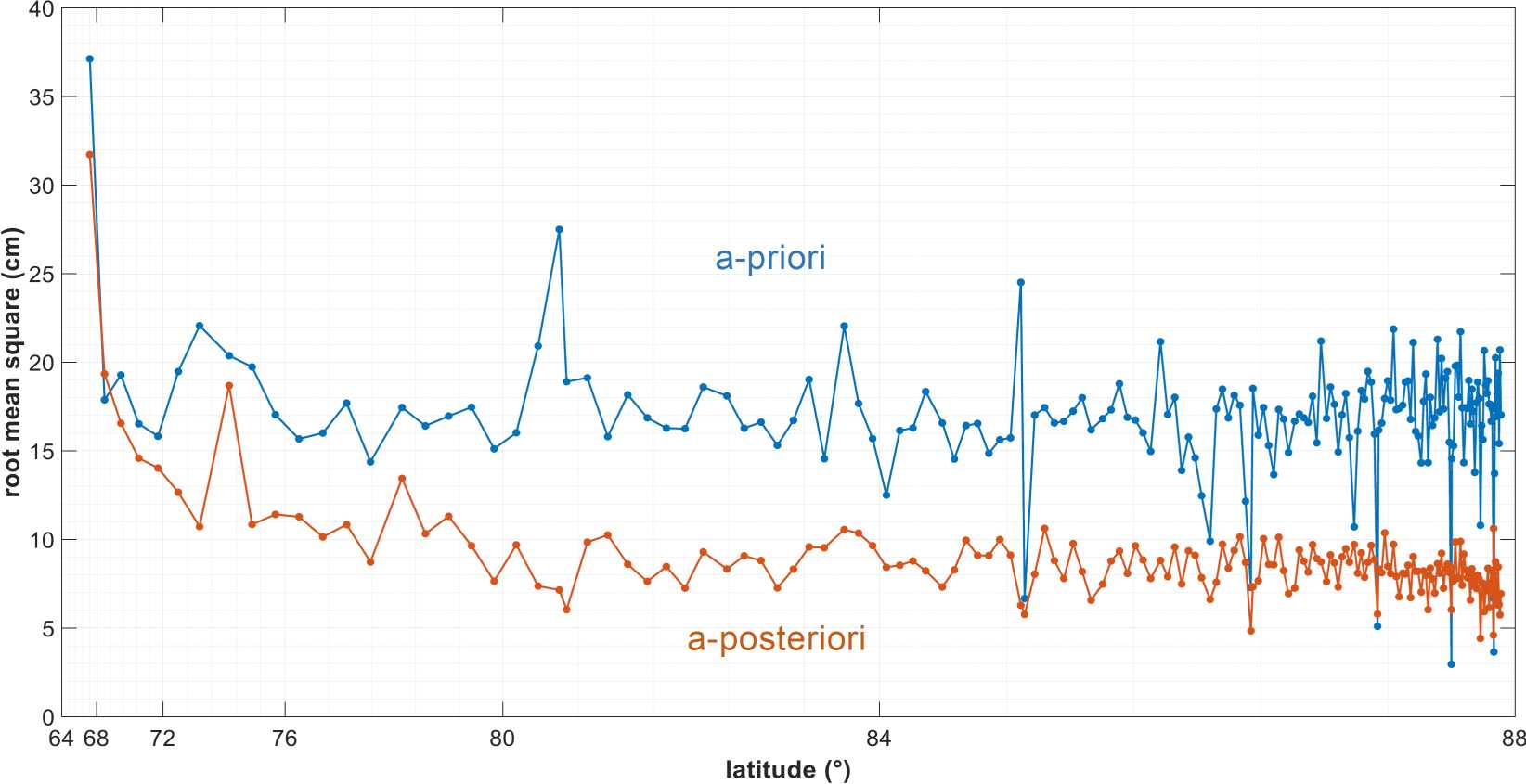# Bo Wang

Crossover Adjustment of ICESat-2 Satellite Altimetry for the Arctic Region

The ICESat-2 mission obtains height measurements to create a global portrait of Earth’s radial dimension, gathering data to monitor changes of terrain including glaciers, sea ice, forests and more. Therefore, it is important to understand the error budget of the observations, one component of which is radial orbit error. Apart from the altimetric ranging errors, radial orbit errors directly influence the precision of the measurement of sea surface height (SSH). These errors can be assessed by analyzing the difference of SSH at ground track intersections, socalled crossover differences (XO differences). An effective approach is to model the orbit error by minimizing the residual XO difference by the least-squares method. XO differences are assumed to be caused by the difference of radial orbit errors between ascending and descending arcs, the sea surface variation, mispointing, and measurement errors. Since the sea surface variation in a short time interval and measurement errors can be considered as random variable, these residuals can be reduced by the method of XO adjustment.

In general, the error function model is described as a function of time or the track length. The most popular parameterization for regional applications is a simple bias or bias-andtilt model. The parameters (bias and tilt) for each track are determined by minimizing the residual XO differences. When the length of a track is a small fraction of one revolution, this parameterization is adequate. However, this is not always the case. For global applications, enhanced parameterizations are to represent the radial orbit error using higher-degree polynomials or Fourier series. Consequently, the choice of parameterization is a balance between efficiency and simplicity. Since the orbits of altimeter satellites are almost circular, at least locally the orbit can be described as a Keplerian orbit. We describe the XO adjustment with Keplerian orbit model in the Arctic region. One reason for our choice of the Arctic region is the fact that ICESat-2 focuses on arctic ocean regions. A second reason is that previous XO analyses chose rectangular and/or diamond shaped regions. Consequently, XO analysis for the Arctic region will help to understand the performance of the LS adjustment over spherical cap geometry. A mathematical function of the radial orbit error is created after linearizing the Keplerian orbit model. One problem of XO adjustment is the existence of singular solutions, which belong to a so-called null space of the normal equations. The situation is comparable to that of a leveling network. Networks of height difference observations are derived by the differential leveling method. A closed leveling network is a loop that begins and ends at one point. Theoretically, the misclosure should be zero, but in fact random errors cannot be avoided, which can be subjected to a least squares adjustment. There will be a datum problem when adjusting the errors, i.e. heights of points can only be determined by fixing the height of one arbitrary point. When a point is fixed, absolute constraints are applied in the adjustment by setting the corresponding parameters to zero. The same holds true here; therefore, we fix parameters falling inside the null space of the XO minimization problem.
The histograms of XO differences before and after adjustment are demonstrated in Figure 1. The RMS is reduced from 17.2 cm to 8.7 cm using the method of the XO adjustment. Assuming the residual errors are independent in the XO difference function, 8.7 cm divided by √2 gives a value of 6.1 cm. That means the orbit error and mispointing error level of ICESat-2 in the Arctic region is generally 6.1 cm.
From Figure 2 we can see XO differences at different latitudes before and after adjustment. At the XOs closest to the North Pole, the differences are reduced to about 6 cm to 7 cm. The reduced value of XO difference divided by √2 gives an approximate value of 4 cm to 5 cm. However, parts of the XO differences are not significantly reduced, which may be caused by the boundaries of sea-ice-land. For this reason, the altimetry will have large measurement errors influenced by these boundaries.Comparison of XO differences at different latitudes before and after adjustment